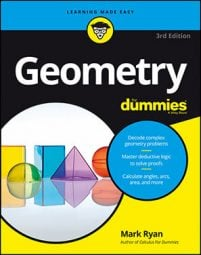##### Geometry For DummiesThere are five ways in which you can prove that a quadrilateral is a parallelogram. The first four are the converses of parallelogram properties (including the definition of a parallelogram). Make sure you remember the oddball fifth one — which isn’t the converse of a property — because it often comes in handy:
• If both pairs of opposite sides of a quadrilateral are parallel, then it’s a parallelogram (reverse of the definition).

• If both pairs of opposite sides of a quadrilateral are congruent, then it’s a parallelogram (converse of a property).

Tip: To get a feel for why this proof method works, take two toothpicks and two pens or pencils of the same length and put them all together tip-to-tip; create a closed figure, with the toothpicks opposite each other. The only shape you can make is a parallelogram.

• If both pairs of opposite angles of a quadrilateral are congruent, then it’s a parallelogram (converse of a property).

• If the diagonals of a quadrilateral bisect each other, then it’s a parallelogram (converse of a property).

Tip: Take, say, a pencil and a toothpick (or two pens or pencils of different lengths) and make them cross each other at their midpoints. No matter how you change the angle they make, their tips form a parallelogram.

• If one pair of opposite sides of a quadrilateral are both parallel and congruent, then it’s a parallelogram (neither the reverse of the definition nor the converse of a property).

Tip: Take two pens or pencils of the same length, holding one in each hand. If you keep them parallel, no matter how you move them around, you can see that their four ends form a parallelogram.

The preceding list contains the converses of four of the five parallelogram properties. If you’re wondering why the converse of the fifth property (consecutive angles are supplementary) isn’t on the list, you have a good mind for details. The explanation, essentially, is that the converse of this property, while true, is difficult to use, and you can always use one of the other methods instead.